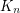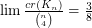Importance: High ✭✭✭
 Author(s):
 Subject: Graph Theory » Topological Graph Theory » » Crossing numbers
 Keywords: complete graph crossing number
 Posted by: Robert Samal on: May 11th, 2007

The crossing numberofis the minimum number of crossings in all drawings ofin the plane.

Conjecture(This discussion appears as [M].)

A drawing of a graphin the plane has the vertices represented by distinct points and the edges represented by polygonal lines joining their endpoints such that:

\item no edge contains a vertex other than its endpoints, \item no two adjacent edges share a point other than their common endpoint, \item two nonadjacent edges share at most one point at which they cross transversally, and \item no three edges cross at the same point.

The conjectured value for the crossing number ofis known to be an upper bound. This is shown by exhibiting a drawing with that number of crossings. If, placevertices regularly spaced along two circles of radii 1 and 2, respectively. Two vertices on the inner circle are connected by a straight line; two vertices on the outer circle are connected by a polygonal line outside the circle. A vertex on the inner circle is connected to one on the outer circle with a polygonal line segment of minimum possible positive winding angle around the cylinder. A simple count shows that the number of crossings in such a drawing achieves the conjectured minimum. Forwe delete one vertex from the drawing described and achieve the conjectured minimum.

The conjecture is known to be true forat most 10 [G]. If the conjecture is true for, then it is also true for. This follows from an argument counting the number of crossings in drawings of all's contained in an optimal drawing of.

It would also be interesting to prove that the conjectured upper bound is asymptotically correct, that is, that.

The best known lower bound is due to Kleitman [K], who showed that this limit is at least.

## Bibliography

[G] R. Guy, The decline and fall of Zarankiewicz's theorem, in Proof Techniques in Graph Theory (F. Harary Ed.), Academic Press, New York (1969) 63-69.

[K] D. Kleitman, The crossing number of, J. Combin. Theory 9 (1970) 315-323.

[M] B. Mohar, Problem of the Month

* indicates original appearance(s) of problem.